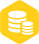# 怎么设计实现一阶数字滤波器

1.1w人已加入

### 描述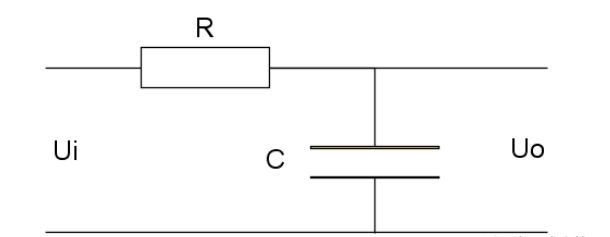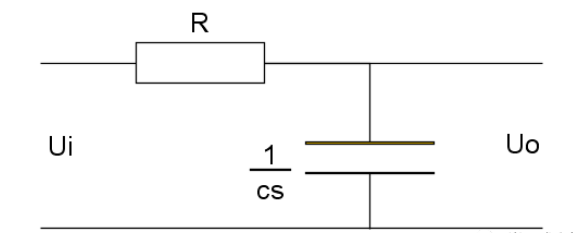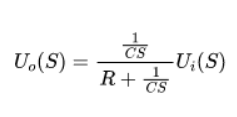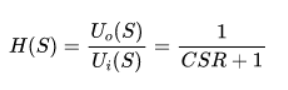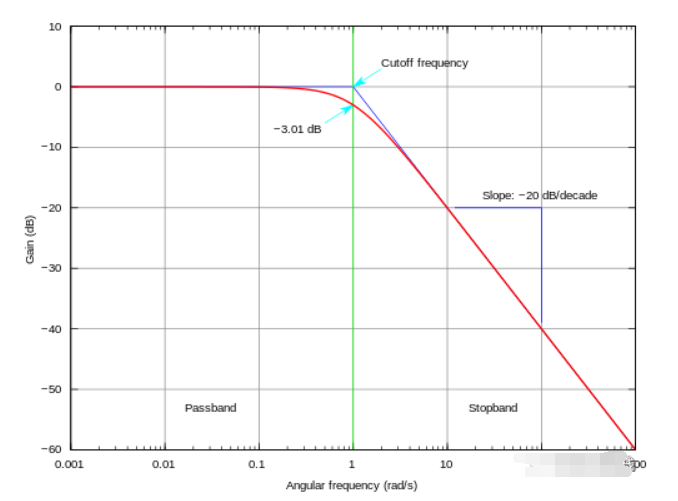http://www.elecfans.com/tools/rclvboqijiezhipinlv.html

MATLAB 代码

clc; format compact s = tf('s'); w = 50; % rad/s H = w/(s+w) T = 1/500; Hd = c2d(H,T,'zoh') opts = bodeoptions; opts.FreqUnits = 'rad/s'; opts.XLim = [0.01, 10000]; opts.Grid = 'on'; bode(H,Hd, opts)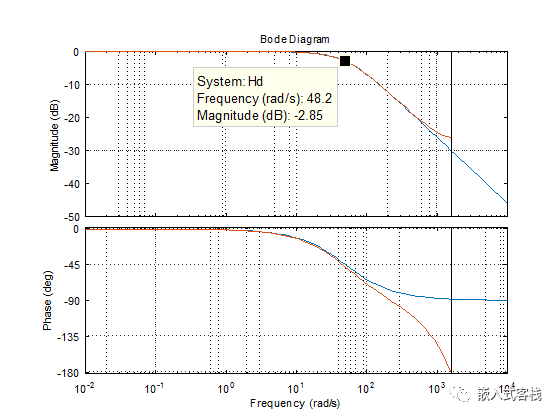#include  #include  #include  typedef struct _t_FSTO_FILTER {     float yn1;     float a; }t_FSTO_FILTER; int init_first_order_lpf(t_FSTO_FILTER * pFilter,float Tf,float T) {     if(T<=0 || Tf<=0)       return -1;     pFilter->a   = Tf/(Tf+T);     pFilter->yn1 = 0;     return 0; } float fist_order_lpf(t_FSTO_FILTER * pFilter,float xn) {     float yn;     yn = pFilter->a*pFilter->yn1+(1-pFilter->a)*xn;     pFilter->yn1 = yn;     return yn; } #define PI 3.1415f #define SAMPLE_RATE 500.0f #define SAMPLE_T (1/SAMPLE_RATE) #define SAMPLE_SIZE (100) int main() {     float sim[SAMPLE_SIZE];     float out[SAMPLE_SIZE];     t_FSTO_FILTER lpf;     if(init_first_order_lpf(&lpf,0.005,SAMPLE_T)==-1)       return -1;     FILE *pFile=fopen("./simulationSin.csv","wt+");     if(pFile==NULL)     {         printf("simulationSin.csv opened failed");         return -1;     }     for(int i=0;i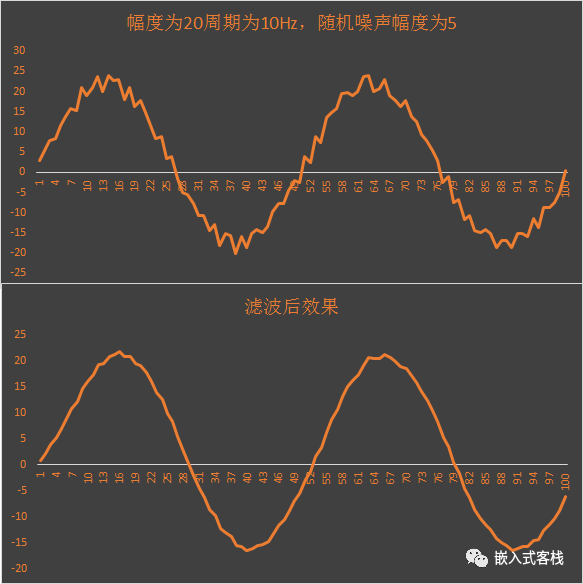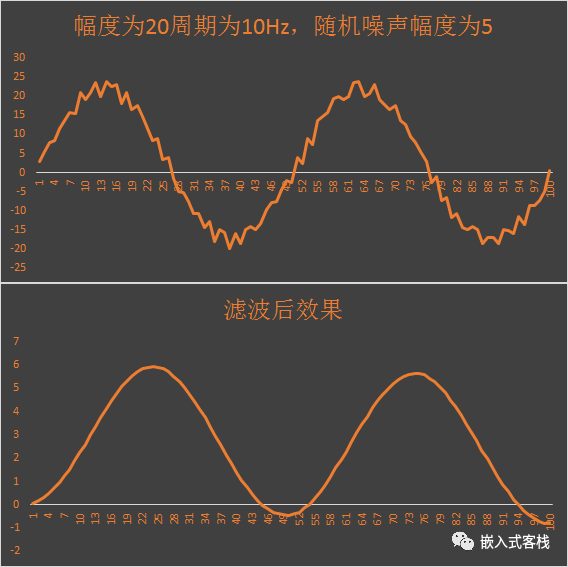×
20